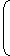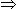# Trains Shortcut Methods

Problems on trains are most frequently asked questions in any competitive exam.
Problems on trains and ‘Time and Distance’ are almost same. The only difference is we have to consider the length of the train while solving problems on trains.
Points To Remember
1. Time taken by a train of length of L meters to pass a stationary pole is equal to the time taken by train to cover L meters.
2. Time taken by a train of length of L meters to pass a stationary object of length P meters is equal to the time taken by train to cover (L + P) meters.
3. If two trains are moving in same direction and their speeds are x km/h and y km/h (x > y) then their relative speed is (x – y) km/h.
4. If two trains are moving in opposite direction and their speeds are x km/h and y km/h then their relative speed is (x + y) km/h.
Some Shortcut Methods

Rule 1:
If two trains of p meters and q meters are moving in same direction at the speed of x m/s and y m/s (x > y) respectively then time taken by the faster train to overtake slower train is given by

see example:
Two trains of length 130 meter and 70 meter are running in the same direction with the speed of 50 km/h and 70 km/h. How much time will faster train take to overtake the slower train from the moment they meet?
Sol:

Let’s say p = 130 meter = 0. 13 km
q = 70 meter = 0.07 km
x = 70 km/h and y = 50 km/h,
So from the equation given above,0.01 hours = 36 second
So it will take 36 seconds to overtake.
Rule 2:

If two trains of p meters and q meters are moving in opposite direction at the speed of x m/s and y m/s respectively then time taken by trains to cross each other is given by

see example
Two trains of length 130 meter and 70 meter are running in the opposite direction with the speed of 50 km/h and 70 km/h. How much time will trains take to cross each other from the moment they meet?
Sol:

Let’s say p = 130 meter = 0. 13 km
q = 70 meter = 0.07 km
x = 70 km/h and y = 50 km/h,
So from the equation given above,0.0017 hours = 6 seconds
So it will take 6 seconds to cross each other.

example:

A train 125 m long passes a man, running at 5 km/hr in the same direction in which the train is going, in 10 seconds. The speed of the train is:

Sol:
 Speed of the train relative to man =125m/sec 10
 =25m/sec. 2
 =25 x 18km/hr 2 5
= 45 km/hr.
Let the speed of the train be x km/hr. Then, relative speed = (x - 5) km/hr.x - 5 = 45x = 50 km/hr.

example:

Two trains running in opposite directions cross a man standing on the platform in 27 seconds and 17 seconds respectively and they cross each other in 23 seconds. The ratio of their speeds is:

Sol:

Let the speeds of the two trains be x m/sec and y m/sec respectively.
Then, length of the first train = 27x metres,
and length of the second train = 17y metres.27x + 17y = 23 x+ y27x + 17y = 23x + 23y4x = 6yx = 3 . y 2

example:

A train passes a station platform in 36 seconds and a man standing on the platform in 20 seconds. If the speed of the train is 54 km/hr, what is the length of the platform?

Sol:
 Speed =54 x 5m/sec = 15 m/sec. 18
Length of the train = (15 x 20)m = 300 m.
Let the length of the platform be x metres.
 Then, x + 300 = 15 36x + 300 = 540x = 240 m.

#### Unknown

Do You Like This??? Then Hit Subscribe Button. You Will Get Every Post, Which Is Worth Reading